# Convert Currency in Number to Words in MS Word

Some times we need numbers in both numerical format and in words. Here I am going to explain how to use VBA module to convert Number to Words in Microsoft Word.

If you are looking for a method to use in MS - Excel, please check my earlier blog post : Convert Currency in Number to Words (Indian Rupees) - MS Excel

### Step 1: Enable Developer Tab In MS Word

If you can't see the developer tab as like in the below picture, Go to File -> Options -> Customize Ribbon -> Check on 'Devloper' under Main Tabs heading, Developer menu enabling process is explained here.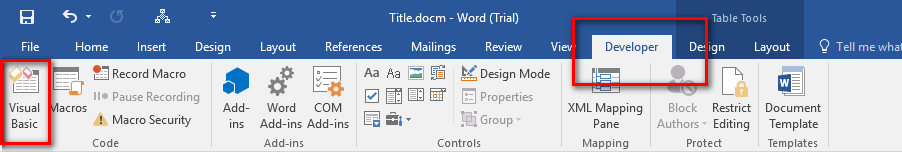### Step 2: Add the Module Code

Click on the Visual Basic option in the Developer Ribbon. Right click on the 'Project' title and Insert -> Module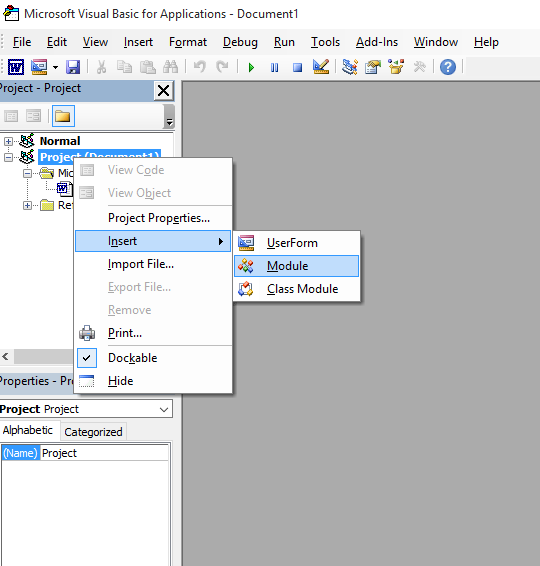Copy and paste the below code in code window of the Module1.

Version 2 adds 'and' before 1st, 10th, 100th and decimal place

``````
Sub ConvertCurrencyToEnglish()
MyNumber = Val(Selection.Text)
Dim Temp
Dim Rupees, Paise
Dim DecimalPlace, Count

ReDim Place(9) As String
Place(2) = " Thousand "
Place(3) = " lakh "
Place(4) = " Crore "

' Convert MyNumber to a string, trimming extra spaces.
MyNumber = Trim(Str(MyNumber))

' Find decimal place.
DecimalPlace = InStr(MyNumber, ".")

' If we find decimal place...
If DecimalPlace > 0 Then
' Convert Paise
Temp = Left(Mid(MyNumber, DecimalPlace + 1) & "00", 2)
' Hi! Note the above line Mid function it gives right portion
' after the decimal point
'if only . and no numbers such as 789. accures, mid returns nothing
' to avoid error we added 00
' Left function gives only left portion of the string with specified places here 2

Paise = ConvertTens(Temp)

' Strip off paise from remainder to convert.
MyNumber = Trim(Left(MyNumber, DecimalPlace - 1))
End If

Count = 1
If MyNumber <> "" Then

' Convert last 3 digits of MyNumber to Indian Rupees.
Temp = ConvertHundreds(Right(MyNumber, 3), Paise, MyNumber)

If Temp <> "" Then Rupees = Temp & Place(Count) & Rupees

If Len(MyNumber) > 3 Then
' Remove last 3 converted digits from MyNumber.
MyNumber = Left(MyNumber, Len(MyNumber) - 3)
Else
MyNumber = ""
End If

End If

' convert last two digits to of mynumber
Count = 2

Do While MyNumber <> ""
Temp = ConvertTens(Right("0" & MyNumber, 2))

If Temp <> "" Then Rupees = Temp & Place(Count) & Rupees
If Len(MyNumber) > 2 Then
' Remove last 2 converted digits from MyNumber.
MyNumber = Left(MyNumber, Len(MyNumber) - 2)

Else
MyNumber = ""
End If
Count = Count + 1

Loop

' Clean up rupees.
Select Case Rupees
Case ""
Rupees = ""
Case "One"
Rupees = "Rupee One"
Case Else
Rupees = "Rupees " & Rupees
End Select

' Clean up paise.
Select Case Paise
Case ""
Paise = ""
Case "One"
Paise = "One Paise"
Case Else
Paise = Paise & " Paise"
End Select

If Rupees = "" Then
Result = Paise & " Only"
ElseIf Paise = "" Then
Result = Rupees & " Only"
Else
Result = Rupees & " and " & Paise & " Only"
End If
Selection.Text = Result

End Sub

Private Function ConvertDigit(ByVal MyDigit)
Select Case Val(MyDigit)
Case 1: ConvertDigit = "One"
Case 2: ConvertDigit = "Two"
Case 3: ConvertDigit = "Three"
Case 4: ConvertDigit = "Four"
Case 5: ConvertDigit = "Five"
Case 6: ConvertDigit = "Six"
Case 7: ConvertDigit = "Seven"
Case 8: ConvertDigit = "Eight"
Case 9: ConvertDigit = "Nine"
Case Else: ConvertDigit = ""
End Select

End Function

Private Function ConvertHundreds(ByVal MyNumber, ByVal Paise, ByVal OriginalNumber)
Dim Result As String

Result10or1 = ""
Result100 = ""

' Exit if there is nothing to convert.
If Val(MyNumber) = 0 Then Exit Function

' Append leading zeros to number.
MyNumber = Right("000" & MyNumber, 3)

' Do we have a hundreds place digit to convert?
If Left(MyNumber, 1) <> "0" Then
Result100 = ConvertDigit(Left(MyNumber, 1)) & " Hundred "
End If

' Do we have a tens place digit to convert?
If Mid(MyNumber, 2, 1) <> "0" Then
Result10or1 = ConvertTens(Mid(MyNumber, 2))
Else
' If not, then convert the ones place digit.
Result10or1 = ConvertDigit(Mid(MyNumber, 3))
End If

' if 1st & 10th places are zero, place 'and' before hundreds
' else place before 10th place

'if paise not exists add 100
If (Paise <> "") Then
Result = Result100 + Result10or1
Else
If (Result100 <> "") Then
If (Result10or1 <> "") Then
Result = Result100 + "and " + Result10or1
Else
If (Len(OriginalNumber) > 3) Then
Result = "and " + Result100
Else
Result = Result100
End If
End If

Else
If (Result10or1 <> "") Then
If (Len(OriginalNumber) > 2) Then
Result = "and " + Result10or1
Else
Result = Result10or1
End If
End If

End If

End If

ConvertHundreds = Trim(Result)
End Function

Private Function ConvertTens(ByVal MyTens)
Dim Result As String

' Is value between 10 and 19?
If Val(Left(MyTens, 1)) = 1 Then
Select Case Val(MyTens)
Case 10: Result = "Ten"
Case 11: Result = "Eleven"
Case 12: Result = "Twelve"
Case 13: Result = "Thirteen"
Case 14: Result = "Fourteen"
Case 15: Result = "Fifteen"
Case 16: Result = "Sixteen"
Case 17: Result = "Seventeen"
Case 18: Result = "Eighteen"
Case 19: Result = "Nineteen"
Case Else
End Select
Else
' .. otherwise it's between 20 and 99.
Select Case Val(Left(MyTens, 1))
Case 2: Result = "Twenty "
Case 3: Result = "Thirty "
Case 4: Result = "Forty "
Case 5: Result = "Fifty "
Case 6: Result = "Sixty "
Case 7: Result = "Seventy "
Case 8: Result = "Eighty "
Case 9: Result = "Ninety "
Case Else
End Select

' Convert ones place digit.
Result = Result & ConvertDigit(Right(MyTens, 1))
End If

ConvertTens = Trim(Result)
End Function

``````

Version 1 adds 'and' only before decimal place (paise)

``````
Sub ConvertCurrencyToEnglish()
MyNumber = Val(Selection.Text)

Dim Temp
Dim Rupees, Paise
Dim DecimalPlace, Count
ReDim Place(9) As String
Place(2) = " Thousand "
Place(3) = " lakh "
Place(4) = " Crore "

' Convert MyNumber to a string, trimming extra spaces.
MyNumber = Trim(Str(MyNumber))

' Find decimal place.
DecimalPlace = InStr(MyNumber, ".")

' If we find decimal place...
If DecimalPlace > 0 Then
' Convert Paise
Temp = Left(Mid(MyNumber, DecimalPlace + 1) & "00", 2)
' Hi! Note the above line Mid function it gives right portion
' after the decimal point
'if only . and no numbers such as 789. accures, mid returns nothing
' to avoid error we added 00
' Left function gives only left portion of the string with specified places here 2

Paise = ConvertTens(Temp)

' Strip off paise from remainder to convert.
MyNumber = Trim(Left(MyNumber, DecimalPlace - 1))
End If

Count = 1
If MyNumber <> "" Then

' Convert last 3 digits of MyNumber to Indian Rupees.
Temp = ConvertHundreds(Right(MyNumber, 3))

If Temp <> "" Then Rupees = Temp & Place(Count) & Rupees

If Len(MyNumber) > 3 Then
' Remove last 3 converted digits from MyNumber.
MyNumber = Left(MyNumber, Len(MyNumber) - 3)
Else
MyNumber = ""
End If

End If

' convert last two digits to of mynumber
Count = 2

Do While MyNumber <> ""
Temp = ConvertTens(Right("0" & MyNumber, 2))

If Temp <> "" Then Rupees = Temp & Place(Count) & Rupees
If Len(MyNumber) > 2 Then
' Remove last 2 converted digits from MyNumber.
MyNumber = Left(MyNumber, Len(MyNumber) - 2)

Else
MyNumber = ""
End If
Count = Count + 1

Loop

' Clean up rupees.
Select Case Rupees
Case ""
Rupees = ""
Case "One"
Rupees = "One Rupee"
Case Else
Rupees = Rupees & " Rupees"
End Select

' Clean up paise.
Select Case Paise
Case ""
Paise = ""
Case "One"
Paise = "One Paise"
Case Else
Paise = Paise & " Paise"
End Select

If Rupees = "" Then
Result = Paise
ElseIf Paise = "" Then
Result = Rupees
Else
Result = Rupees & " and " & Paise
End If
Selection.Text = Result

End Sub

Private Function ConvertDigit(ByVal MyDigit)
Select Case Val(MyDigit)
Case 1: ConvertDigit = "One"
Case 2: ConvertDigit = "Two"
Case 3: ConvertDigit = "Three"
Case 4: ConvertDigit = "Four"
Case 5: ConvertDigit = "Five"
Case 6: ConvertDigit = "Six"
Case 7: ConvertDigit = "Seven"
Case 8: ConvertDigit = "Eight"
Case 9: ConvertDigit = "Nine"
Case Else: ConvertDigit = ""
End Select

End Function

Private Function ConvertHundreds(ByVal MyNumber)
Dim Result As String

' Exit if there is nothing to convert.
If Val(MyNumber) = 0 Then Exit Function

' Append leading zeros to number.
MyNumber = Right("000" & MyNumber, 3)

' Do we have a hundreds place digit to convert?
If Left(MyNumber, 1) <> "0" Then
Result = ConvertDigit(Left(MyNumber, 1)) & " Hundred "
End If

' Do we have a tens place digit to convert?
If Mid(MyNumber, 2, 1) <> "0" Then
Result = Result & ConvertTens(Mid(MyNumber, 2))
Else
' If not, then convert the ones place digit.
Result = Result & ConvertDigit(Mid(MyNumber, 3))
End If

ConvertHundreds = Trim(Result)
End Function

Private Function ConvertTens(ByVal MyTens)
Dim Result As String

' Is value between 10 and 19?
If Val(Left(MyTens, 1)) = 1 Then
Select Case Val(MyTens)
Case 10: Result = "Ten"
Case 11: Result = "Eleven"
Case 12: Result = "Twelve"
Case 13: Result = "Thirteen"
Case 14: Result = "Fourteen"
Case 15: Result = "Fifteen"
Case 16: Result = "Sixteen"
Case 17: Result = "Seventeen"
Case 18: Result = "Eighteen"
Case 19: Result = "Nineteen"
Case Else
End Select
Else
' .. otherwise it's between 20 and 99.
Select Case Val(Left(MyTens, 1))
Case 2: Result = "Twenty "
Case 3: Result = "Thirty "
Case 4: Result = "Forty "
Case 5: Result = "Fifty "
Case 6: Result = "Sixty "
Case 7: Result = "Seventy "
Case 8: Result = "Eighty "
Case 9: Result = "Ninety "
Case Else
End Select

' Convert ones place digit.
Result = Result & ConvertDigit(Right(MyTens, 1))
End If

ConvertTens = Result
End Function

``````

You can also rename the project title (Right click on the 'Project' title, Project Properties, provide a name you wish. For example 'MyWordTools')

### Step 3: Create a shortcut button to run the Macro

Click on the customize quick access button in MS Word Window top bar and chose 'More Commands' option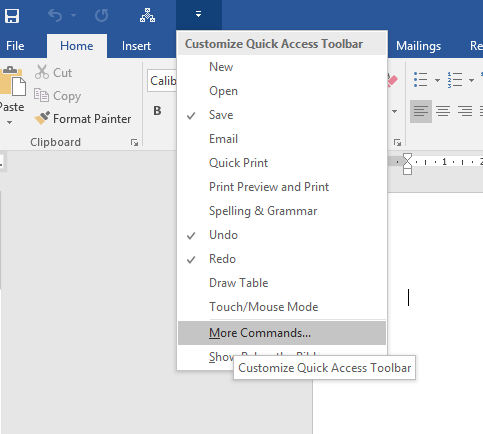Now the Word options window will appear as like below.

Choose Macros from the 'Choose commands from' combo box.

Double click on the our custom module Name, now the icon will be added to the right side. Click 'Ok' to save and close.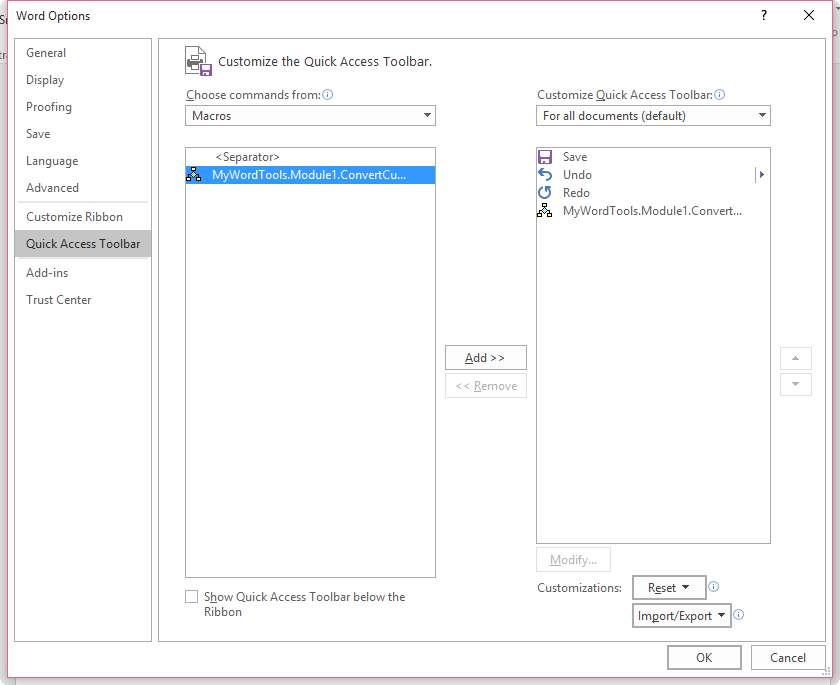Now save the document as 'Macro Enabled Word document' (*.docm)

Now select the number in your document, then click on the button in the quick access bar, the number will be converted into words.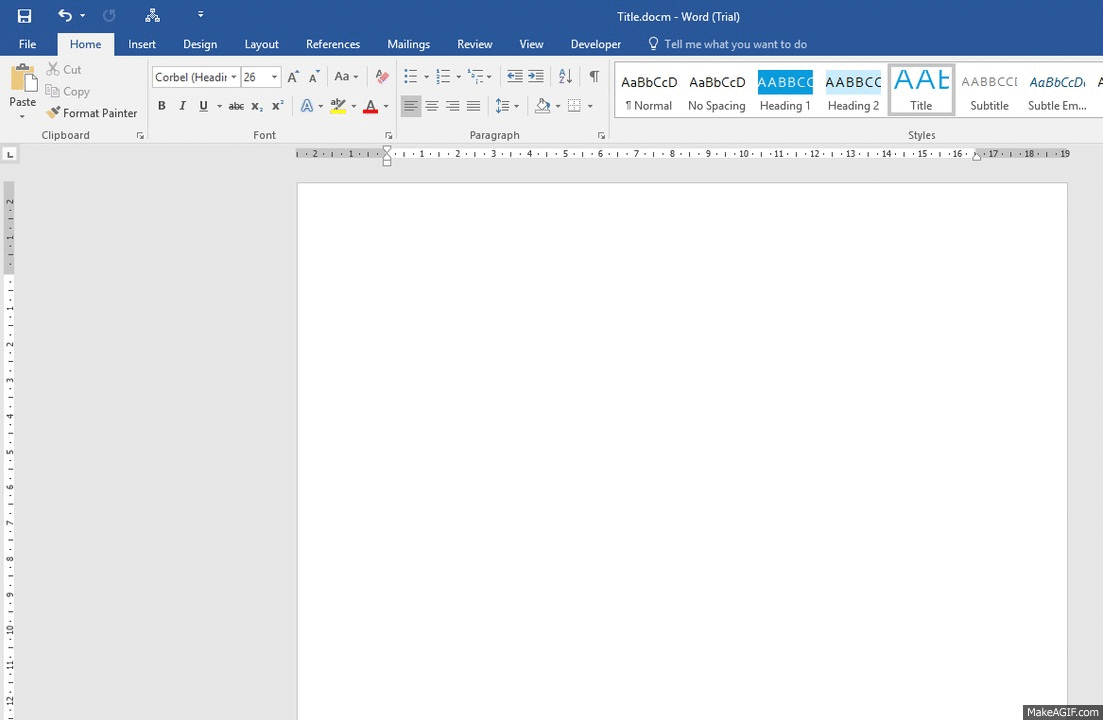### Demo video

Category

Karthikeyan

Aug 20, 2020 - 19:02

In reply to by Shashikant Ganpule

Hi, this code written for typical cheque writing / printing where we do not mention the zero paise and there is no chance of negative numbers. But, the code can be altered easily.
For adding 'Minus' you can use the formula like the one below,
=if(A1<0, "Minus ","") & ConvertCurrencyToEnglish(A1)

Ajith Kumar Pa…

Aug 20, 2020 - 18:18

in the MS Word document, need to come number to word in two different columns one column for number and other one columns for words. kindly help to me how to do it. let my know any other code for that.

Shashikant Ganpule

Oct 16, 2019 - 09:58

I have found very useful . One request to you for little modification . 1) Zero is required ( means : supposed if it is 4.00 then Four Rupees and Zero paise Or 00.73 Zero Rupees and Seventy Three Paise Required 2) Negative number is not taken into consideration . -37.56 ( Minus Thirty Seven Rupee and Fifty Six Paise

Jun 11, 2019 - 14:40

Hi, Broken code fixed. Thanks for notifying us.

Jun 11, 2019 - 12:37

unable to copy the code here. It seems broken to me.

Ajay

Aug 21, 2018 - 16:16

Ajay

Aug 21, 2018 - 15:08

Partho Biswas

Jun 12, 2018 - 12:14

Can you please rectify the code because the term "Rupees" should come first.

Karthikeyan

Oct 26, 2017 - 19:31

In reply to by Sushil Kumar

If it is in Excel, you can use this function as formula to convert multiple figures.

Sushil Kumar

Oct 26, 2017 - 11:56

HI, can i convert multiple figures within one click with the help of this macro

Karthikeyan

Jul 22, 2017 - 17:07

Yes, you can use it in MS Excel 2007.

Sham

Jul 19, 2017 - 17:42

thnx
done

Sham

Jul 19, 2017 - 17:36

please suggest, i m able to use this command in ms excel 2007

Sham

Jul 19, 2017 - 17:35

Karthikeyan

Jul 15, 2017 - 16:36

Custom code needs run macro otherwise Microsoft provides native support to convert number to words.

Antonio

Jun 22, 2017 - 10:16

Hello, great tutorial.
Isn't there a way to do that without running the macro? I mean, like you do in MS Excel.
Thanks

Saketh Sharath Babu

Feb 05, 2017 - 08:02

Very usefull Article bro, but can updaet us with a code without paise?
Kindly Help
regards.

B.K.Reddy.G

Mar 04, 2016 - 17:12

very useful

Mohamedgani

Feb 15, 2016 - 07:14